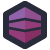Mistakes happen. Errors crop up. Since we know this, we need to defend against it. When it comes to things like account numbers, we can make a rule about which numbers are valid by using a checksum. A simple checksum might be, "Add the digits together, and repeat until you get a single digit, which, after modulus with a constant, must be zero." This means that most simple data-entry errors will result in an invalid account number, but there's still a nice large pool of valid numbers to draw from.

James works for a company that deals with tax certificates, and thus needs to generate numbers which meet a similar checksum rule. Unfortunately for James, this is how his predecessor chose to implement it:

``````while (true)
{
digits = "";
for (int i = 0; i < certificateNumber.ToString().Length; i++)
{
int doubleDigit = Convert.ToInt32(certificateNumber.ToString().Substring(i, 1)) * 2;
digits += (doubleDigit.ToString().Length > 1 ?
Convert.ToInt32(doubleDigit.ToString().Substring(0, 1)) + Convert.ToInt32(doubleDigit.ToString().Substring(1, 1))
: Convert.ToInt32(doubleDigit.ToString().Substring(0, 1)));
}
int result = digits.ToString().Sum(c => c - '0');

if ((result % 10) == 0)
break;
else
certificateNumber++;
}
``````

Whitespace added to make the ternary vaguely more readable.

We start by treating the number as a string, which allows us to access each digit individually, and as we loop, we'll grab a digit and double it. That, unfortunately, gives us a number, which is a big problem. There's absolutely no way to tell if a number is two digits long without turning it back into a string. Absolutely no way! So that's what we do. If the number is two digits, we'll split it back up and add those digits together.

Which again, gives us one of those pesky numbers. So once we've checked every digit, we'll convert that number back to a useful string, then `Sum` the characters in the string to produce a result. A result which, we hope, is divisible by 10. If not, we check the next number. Repeat and repeat until we get a valid result.

The worst part is, though, is that you can see from the `while` loop that this is just dropped into a larger method. This isn't a single function which generates valid certificate numbers. This is a block that gets dropped in line. Similar, but slightly different blocks are dropped in when numbers need to be validated. There's no single `isValidCertificate` method.[Advertisement] Continuously monitor your servers for configuration changes, and report when there's configuration drift. Get started with Otter today!